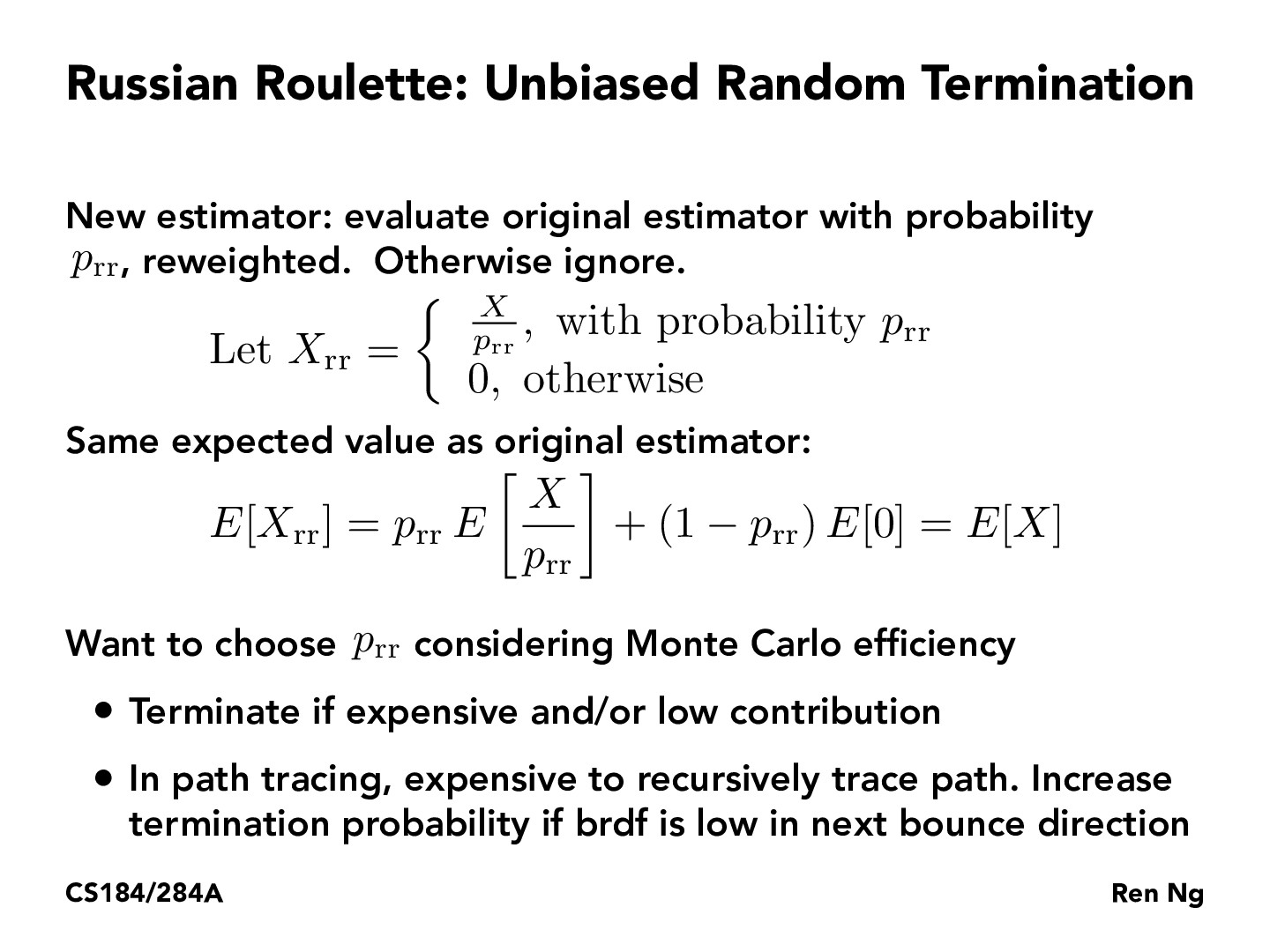You are viewing the course site for a past offering of this course. The current offering may be found here.Lecture 13: Global Illumination and Path Tracing (81)JerryJiehanWang

Here's a good paper about determining the monte carlo efficiency https://www.sciencedirect.com/science/article/pii/0378437194900922tanmayghai18

In terms of Russian Roulette and the added probability coefficient, even after completing the project, I am sort of unsure why it produces an unbiased estimator for random termination?sirejdua

If we want to show that Xrr is an unbiased estimator of X, then we need to show that E[Xrr - X] = 0, that there is no 'bias' between our estimator and the true random variable. Here, we use the definition of expectation to split E[Xrr] = (1-prr)E + prrE[X/prr] = prr/prr*E[X] = E[X]. Linearity of expectation is also used.raghav-cs184

The trick used to define X_rr here is actually just a common indicator function used all over in probability, scaled by a constant. Indicator functions are useful because they let us control the mean (in this manner) while being easy to interpret, and letting us decompose larger events in terms of these smaller indicators.keirp

If we terminate then is it really unbiased?dtseng

^I think if we terminate early by setting a maximum number of bounces, then it's definitely biased. However, if you check out slides 62 and 63, you can see that the difference isn't really that big between 8 bounces and 16 bounces (you get diminishing returns in terms of accurate representation of the scene). So while terminating early is biased, if the maximum number of bounces is set high enough, then the bias is minimal

You must be enrolled in the course to comment